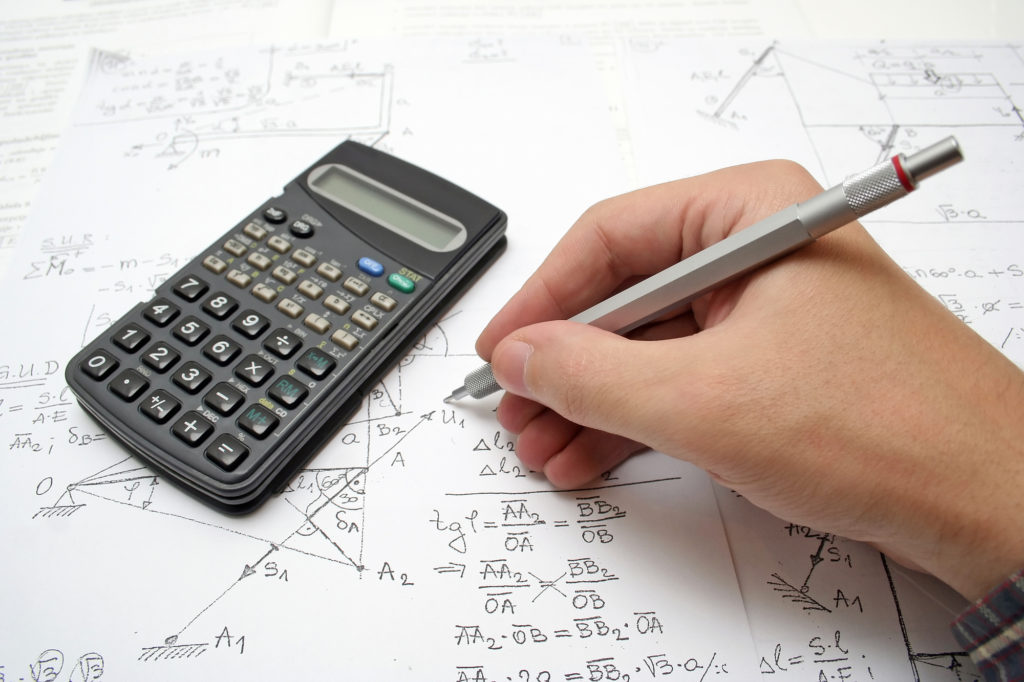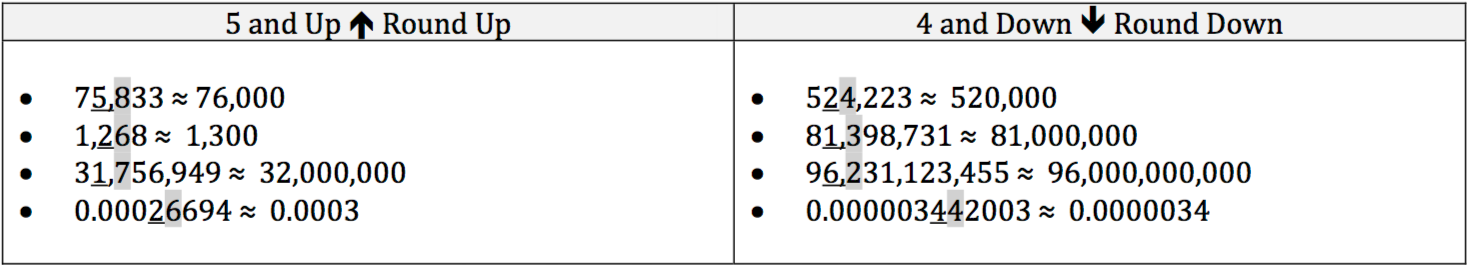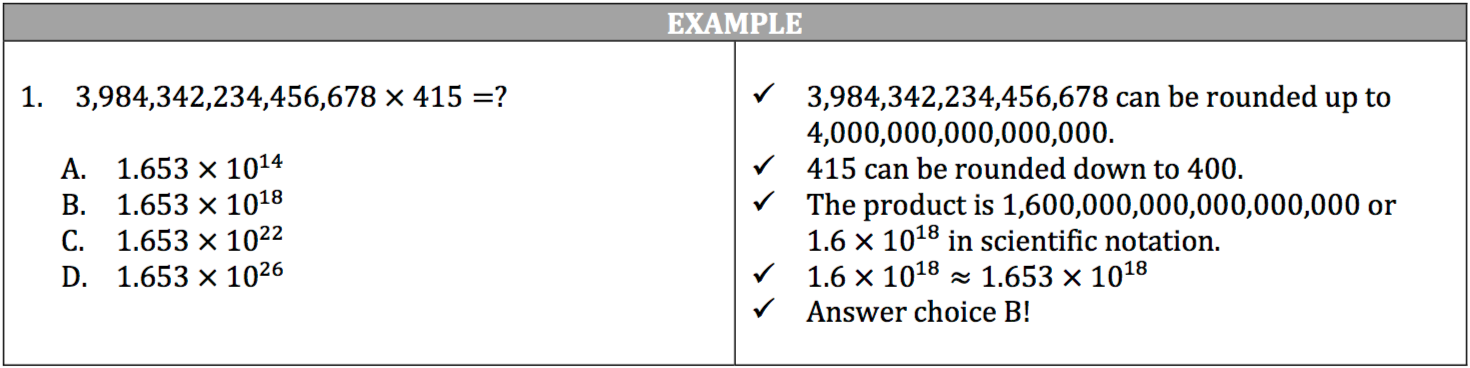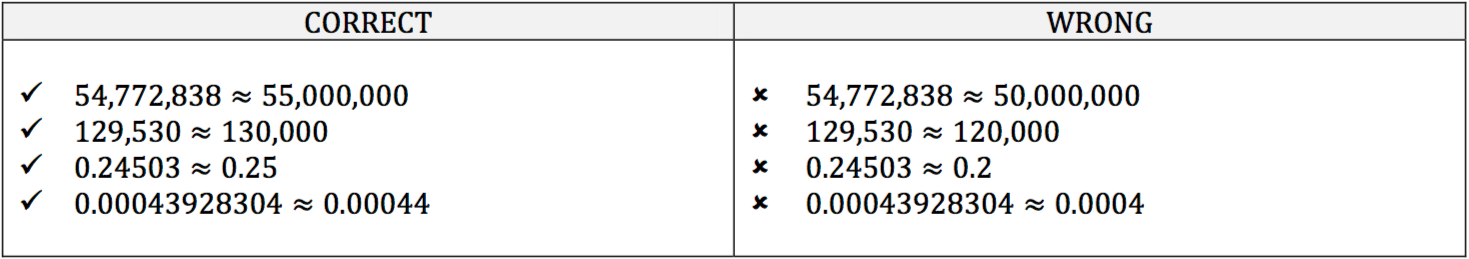For the ISEE and other standardized tests with math sections, estimation is your friend. Estimation will reduce the amount of time you spend making calculations, which allows more time to complete difficult questions.

Estimation is rounding a number up or down to make it easier to calculate, but the estimated number must be as close in value to the original number as possible. In general, you choose a specific place value to estimate, using the next lowest place value as your guide. If the next lowest place value is 5 or greater, you round that place value up. If the next lowest place value is 4 or lower, you round that place value down.## Estimation and Rounding Example

For example, you are asked to find the product of 3,984,342,234,456,678 and 415. Without a calculator, you know this will take you ages to multiply. You can estimate these values:When you think about the original number’s value, look at the three highest place values. Think about how much your estimation will change the original number. If the estimation of the highest place value results in a very different number, look to the next highest place value.

Generally, estimating the second highest place value will result in a number that is truer to the essence of the original.